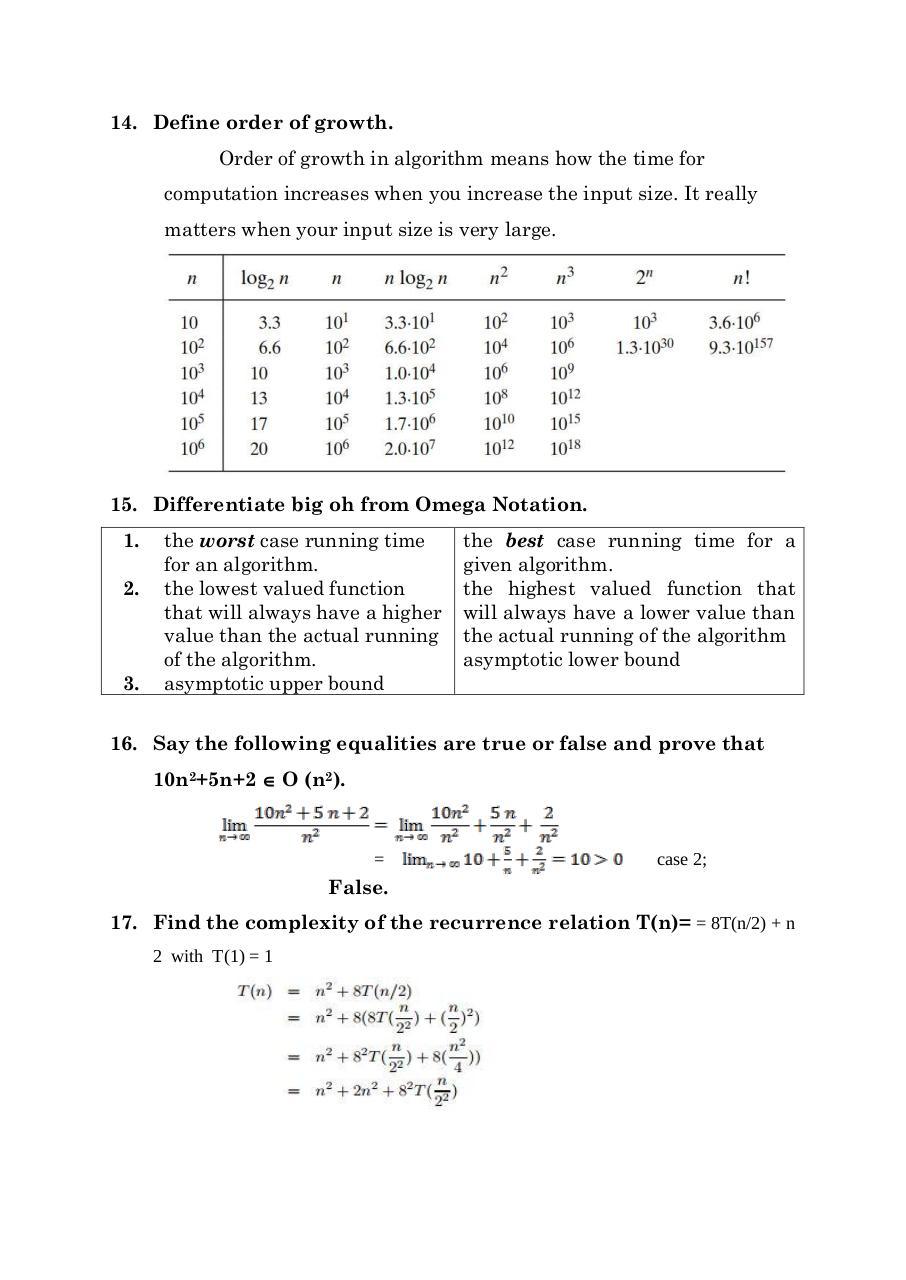# UNIT 1 QB.pdfPage 1 2 3 4 5 6 7 8

#### Text preview

14. Define order of growth.
Order of growth in algorithm means how the time for
computation increases when you increase the input size. It really
matters when your input size is very large.

15. Differentiate big oh from Omega Notation.
1.
2.

3.

the worst case running time
for an algorithm.
the lowest valued function
that will always have a higher
value than the actual running
of the algorithm.
asymptotic upper bound

the best case running time for a
given algorithm.
the highest valued function that
will always have a lower value than
the actual running of the algorithm
asymptotic lower bound

16. Say the following equalities are true or false and prove that
10n2+5n+2  O (n2).

=

case 2;

False.
17. Find the complexity of the recurrence relation T(n)= = 8T(n/2) + n
2 with T(1) = 1